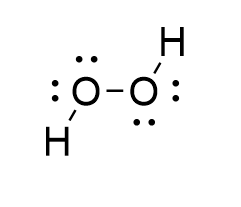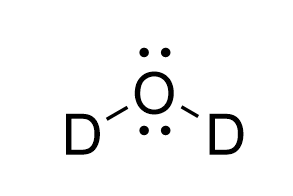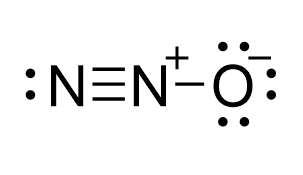Question

# Which of the following is a linear molecule?

A
B
C
D
Medium

## SolutionVerified by Toppr
Correct option is
D

## In , the oxygen atom is the central atom which is surrounded with two hydrogen atoms and hence will              have  two bond pairs. The oxygen atoms will also consist of two lone pairs  in  H_2O\$.             Hence the shape of will be bent and the geometry will be tetrahedral.A molecule consists of two hydrogen atoms and two oxygen atoms. Each oxygen atom in is                surrounded  with two other atoms and two lone pairs. Hence the shape around each oxygen atom                in will be  bent.In , the oxygen atom is the central atom which is surrounded with two deuterium                atoms and hence will have two bond pairs. The oxygen atoms will also consist of two lone                pairs in . Hence the shape of will be bent and the geometry will be tetrahedral., has below structure. The central nitrogen atom is forming two bonds and two                 bonds. So it is hybridized. So the shape is linear..Hence, the correct answer is option .00